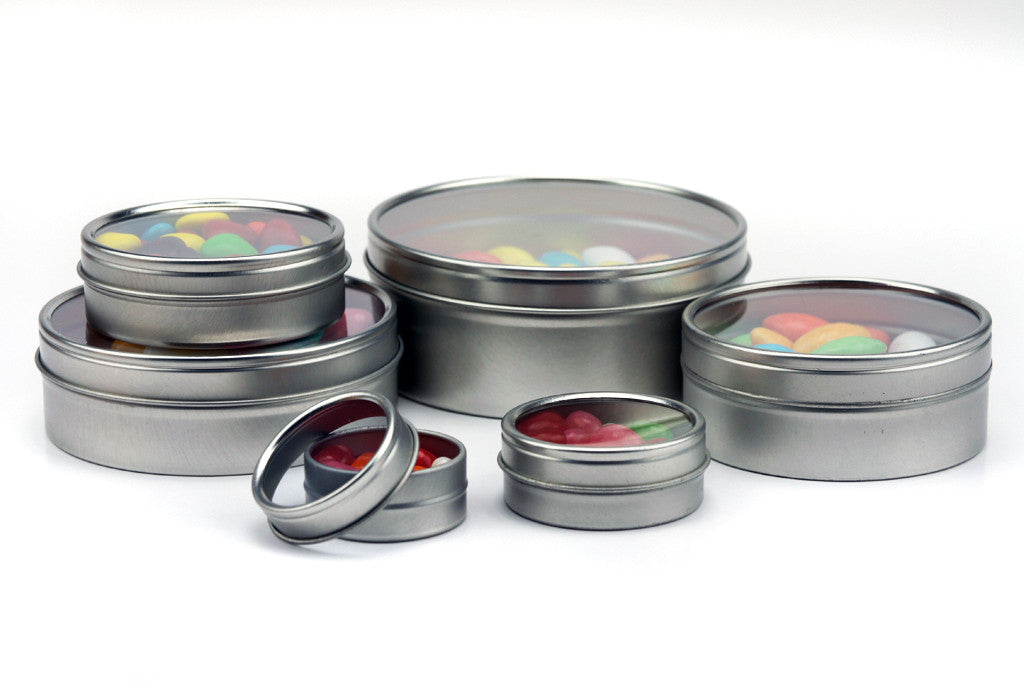Call Us : 626-988-4318

# Silver Round Shallow Seamless Window Top Slip Cover Lid Metal Tin ContainersMimi Pack Silver Round Shallow Seamless Window Top Slip Cover Lid Metal Tin Containers packaging consists of 24 tins. The tins have accurate tracks to guarantee a perfect fit on the twist tops and they are made of plated steel. The bottom and top part of the tins are flawlessly shaped and melded.

Volume(oz) Diameter(mm) Height(mm) Pack Quantity
1/4 34 12 24
1/2 39 17 24
1 50 18 24
2 62 21 24
3 69 21 24
4 80 29 24
6 95 29 24
8 102 30 24
12 111 40 24
16 120 45 24

Hit the "ADD TO CART" button and get yours today!
Size Color Item Number Dimensions
Diameter x Height(inches)
Quantity/Case Case Price(24) Unit Price Add To Cart
Black 1/2oz \$8.78
Liquid error: divided by 0 / unit
Blue 1/2oz \$8.78
Liquid error: divided by 0 / unit
Copper 1/2oz \$8.78
Liquid error: divided by 0 / unit
Gold 1/2oz \$8.78
Liquid error: divided by 0 / unit
Green 1/2oz \$8.78
Liquid error: divided by 0 / unit
Red 1/2oz \$8.78
Liquid error: divided by 0 / unit
Silver 1/2oz \$8.78
Liquid error: divided by 0 / unit
Black 1oz \$10.51
Liquid error: divided by 0 / unit
Blue 1oz \$10.51
Liquid error: divided by 0 / unit
Copper 1oz \$10.51
Liquid error: divided by 0 / unit
Gold 1oz \$10.51
Liquid error: divided by 0 / unit
Green 1oz \$10.51
Liquid error: divided by 0 / unit
Red 1oz \$10.51
Liquid error: divided by 0 / unit
Silver 1oz \$10.51
Liquid error: divided by 0 / unit
Black 2oz \$12.82
Liquid error: divided by 0 / unit
Blue 2oz \$12.82
Liquid error: divided by 0 / unit
Copper 2oz \$12.82
Liquid error: divided by 0 / unit
Gold 2oz \$12.82
Liquid error: divided by 0 / unit
Green 2oz \$12.82
Liquid error: divided by 0 / unit
Red 2oz \$12.82
Liquid error: divided by 0 / unit
Silver 2oz \$14.62
Liquid error: divided by 0 / unit
Black 3oz \$13.68
Liquid error: divided by 0 / unit
Blue 3oz \$13.68
Liquid error: divided by 0 / unit
Copper 3oz \$13.68
Liquid error: divided by 0 / unit
Gold 3oz \$13.68
Liquid error: divided by 0 / unit
Green 3oz \$13.68
Liquid error: divided by 0 / unit
Red 3oz \$13.68
Liquid error: divided by 0 / unit
Silver 3oz \$13.68
Liquid error: divided by 0 / unit
Black 4oz \$17.85
Liquid error: divided by 0 / unit
Blue 4oz \$17.85
Liquid error: divided by 0 / unit
Copper 4oz \$17.85
Liquid error: divided by 0 / unit
Gold 4oz \$17.85
Liquid error: divided by 0 / unit
Green 4oz \$17.85
Liquid error: divided by 0 / unit
Red 4oz \$17.85
Liquid error: divided by 0 / unit
Silver 4oz \$17.85
Liquid error: divided by 0 / unit
Black 6oz \$21.16
Liquid error: divided by 0 / unit
Blue 6oz \$21.16
Liquid error: divided by 0 / unit
Copper 6oz \$21.16
Liquid error: divided by 0 / unit
Gold 6oz \$21.16
Liquid error: divided by 0 / unit
Green 6oz \$21.16
Liquid error: divided by 0 / unit
Red 6oz \$21.16
Liquid error: divided by 0 / unit
Silver 6oz \$21.16
Liquid error: divided by 0 / unit
Black 8oz \$24.62
Liquid error: divided by 0 / unit
Blue 8oz \$24.62
Liquid error: divided by 0 / unit
Copper 8oz \$24.62
Liquid error: divided by 0 / unit
Gold 8oz \$24.62
Liquid error: divided by 0 / unit
Green 8oz \$24.62
Liquid error: divided by 0 / unit
Red 8oz \$24.62
Liquid error: divided by 0 / unit
Silver 8oz \$24.62
Liquid error: divided by 0 / unit
Black 12oz \$27.79
Liquid error: divided by 0 / unit
Blue 12oz \$27.79
Liquid error: divided by 0 / unit
Copper 12oz \$27.79
Liquid error: divided by 0 / unit
Gold 12oz \$27.79
Liquid error: divided by 0 / unit
Green 12oz \$27.79
Liquid error: divided by 0 / unit
Red 12oz \$27.79
Liquid error: divided by 0 / unit
Silver 12oz \$27.79
Liquid error: divided by 0 / unit
Black 16oz \$33.84
Liquid error: divided by 0 / unit
Blue 16oz \$33.84
Liquid error: divided by 0 / unit
Copper 16oz \$33.84
Liquid error: divided by 0 / unit
Gold 16oz \$33.84
Liquid error: divided by 0 / unit
Green 16oz \$33.84
Liquid error: divided by 0 / unit
Red 16oz \$33.84
Liquid error: divided by 0 / unit
Silver 16oz \$33.84
Liquid error: divided by 0 / unit